Editor Ratings:
User Ratings:
[Total: 3 Average: 4.7]

This article includes 5 free online concrete volume calculator websites. You will be able to calculate volume of concrete needed based on concrete depth, width, length, etc. The good thing is you can calculate concrete volume for different shapes like concrete slab, wall, round footings, stairs, etc.

A few unique features are also available that you can use. For example, you can check the sample diagram for concrete calculation, add number of quantity for concrete type, check estimated price per cubic for each concrete quantity, etc.

Let’s check these free online concrete volume calculators.

## CalculatorSoup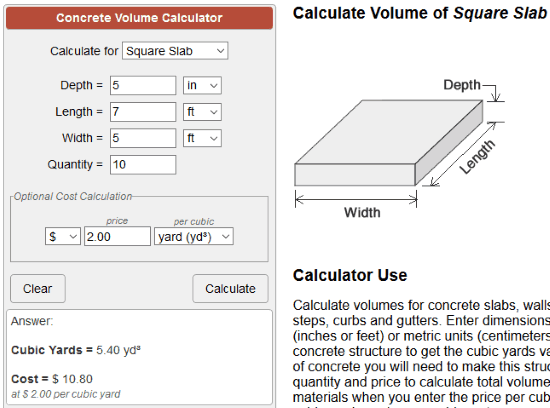CalculatorSoup is one of the best concrete volume calculator websites. It lets you calculate concrete volume for square slab, wall, round column, square column, curb & gutter, footer, etc. For each type of calculation, it also shows a diagram which shows depth, length, and width areas.

This website also brings estimated price calculator for output volume of calculator. You can set the quantity, currency type (dollar, rupee, pound sterling, etc.), price per cubic feet/cubic yard/cubic meter, and it will show the estimated cost.

You can select the option for which you want to calculate the volume of concrete and then add the details. Enter the values for quantity, width, diameter, depth, and length, and get the output. It will show concrete volume in cubic yards.

## Godrejconstruction.com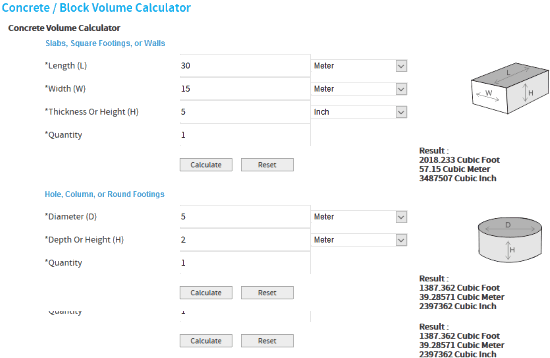Godrejconstruction.com is a good competitor to CalculatorSoup website. It also lets you calculator volume of concrete for:

• Concrete slabs, walls, or square footings.
• Column, hole, or round footings, and
• Tube or circular slab.

Three different sections are available for each calculation. You can provide details in all the sections and then get the results one by one. The output will show you volume of concrete in cubic meter, cubic inch, and cubic feet along with a sample diagram for structure type (square footings, round footings, etc.).

## Calculator.net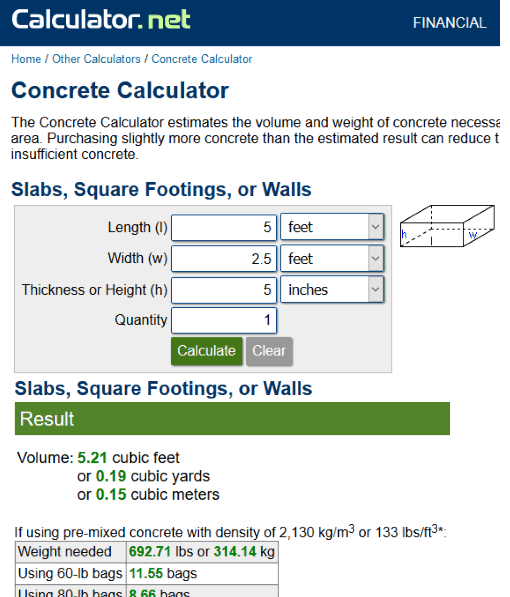Calculator.net lets you calculate estimated volume and weight of concrete to cover different types of areas. It is similar to CalculatorSoup website (mentioned above). Here is the link to its concrete calculator. You can calculate volume and weight required for square footings, circular slab, curb and gutter barrier, stairs, etc.

Provide the input values like area length, width (in yards, inches, feet, centimeters, or meters), material thickness or height, and quantity. Separate calculators are available to calculator concrete volume. Use any calculator and you will get the result. The output will show you concrete volume in cubic yards, cubic feet, or cubic meters.

It also shows if you will use pre-mixed calculate with specified density, then how many 60-lb or 80lb bags of concrete will be needed.

## Calculators.org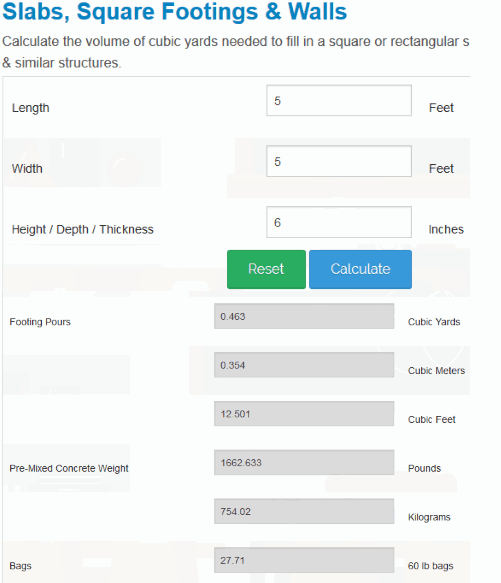Calculators.org is another useful concrete volume calculator website. You can access its Slabs tab where you can add input entries and check the output. Fill the required values like slab or area length and width (in feet) and thickness or height and result will be generated quickly.

The output will include:

• Footing pours or volume of concrete in cubic feet, cubic yards, and cubic meters.
• Pre-mixed concrete weight, and
• Number of bags of concreted needed with 60lb and 80 lb weight.

That’s all this website does for concrete volume calculation.

## Concretenetwork.com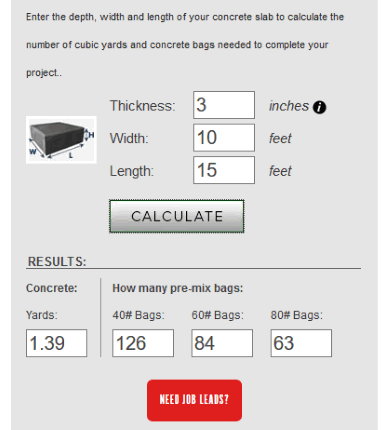Concretenetwork.com is also a nice option to find out volume of concrete and number of pre-mix bags needed based on the output volume. It lets you calculate volume of concrete for slabs, footings, and columns. Three different tabs are available for each type of calculation.

Use a tab and provide input like concrete thickness, width, height, etc., and generate the result. The output will show your concrete volume in yards and how many bags are needed for 40 lb, 80 lb, and 60 lb.

### The Conclusion:

Here I close this list which has best free online concrete volume calculator websites. Among all these online concrete volume calculators, the first website is more useful. It is so because it also comes with estimated price calculator feature.

 Editor Ratings: User Ratings:[Total: 3 Average: 4.7] Tags: calculator